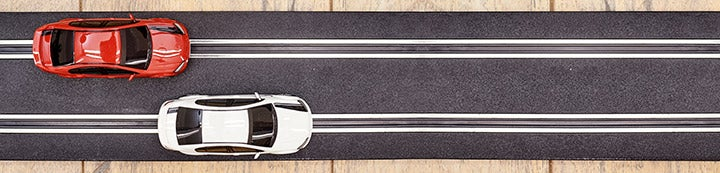1 - 2 of 2 Posts

RichD

·
Rich Dumas
Joined
·
3,813 Posts
Nichrome wire is what is used for controllers. Putting a fixed resistor in parallel with the variable one will lower the overall resistance, but the combination will not have a linear response. I would go for a Parma controller.

RichD

·
Rich Dumas
Joined
·
3,813 Posts
Blame it on Ohm's Law! When two resistors are connected in parallel the reciprocal value of the effective resistance is equal to the sum of the reciprocal values of the parallel resistors. 1/RE = 1/R1 + 1/R2
Suppose that we try to make a 65 ohm controller act like a 25 ohm controller. RE = 25 and R1 = 65 in that case. 1/R2 = 1/25 - 1/65. Therefore R2 = 40.65 ohms. The problem crops up when you squeeze the trigger more. See the chart for a comparison of 25 ohm, 65 ohm and 65 ohm with a parallel 40.65 ohm fixed resistor.

The 25 ohm controller and the modified 65 ohm controller are clearly not the same. The effect is not as drastic if you do not try to lower the effective resistance too much. I shall point out that some people like controllers like that. You should give this idea a try and see how you like it.

1 - 2 of 2 Posts